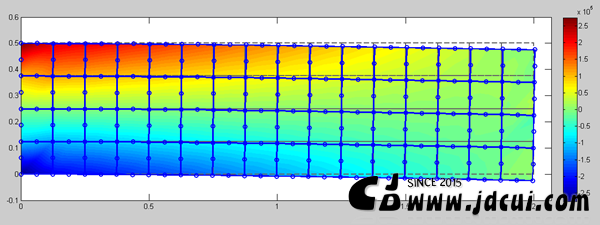•. 2D Truss by Matlab [Matlab 2D桁架有限元] (code by myself)

. 3D Truss by MATLAB[MATLAB 3D 桁架静力分析] (code by myself)

. [FEM][有限元][编程][Matlab][Code by myself] FEM Analysis: 2D Truss Element [有限元分析: 2D桁架单元]

. [FEM][有限元][编程][Matlab][Code by myself] FEM Analysis: 2D Euler Beam Element [有限元分析: 2D欧拉梁单元]

. [FEM][有限元][编程][Matlab][Code by myself] 2D剪切梁单元

. [FEM][有限元][编程][Matlab][Code by myself] 2D Timoshenko梁单元

. [FEM][有限元][编程][Matlab][Code by myself] 三角形常应变单元(CST)

. [FEM][有限元][编程][Matlab][Code by myself] 平面4变形单元(Q4)

. [FEM][有限元][编程][Matlab][Code by myself] 4节点四面体单元(TET4)

. [FEM][有限元][编程][Matlab][Code by myself] 8节点六面体单元(C3D8)

. [FEM][有限元][编程][Matlab][Code by myself] 平面8节点二次“完全积分”单元(CPS8)

. [FEM][有限元][编程][Matlab][Code by myself] 平面6节点二次“完全积分”单元(CPS6)

. [FEM][MATLAB][有限元] FEM Modal Analysis Programming with MATLAB (Truss Element) (桁架单元模态分析编程)

. [FEM][MATLAB][有限元] FEM Modal Analysis Programming with MATLAB (Frame Elements) (框架单元模态分析编程)

. [力学][有限元]Basics of Linear Buckling Analysis [线性曲屈分析基础]

. [FEM][MATLAB][有限元][编程] FEM Buckling Analysis Programming with MATLAB (Truss Elements) (桁架单元曲屈分析编程)

. [FEM][MATLAB][有限元][编程] FEM Buckling Analysis Programming with MATLAB (Frame Elements) (框架单元曲屈分析编程)

This site uses Akismet to reduce spam. Learn how your comment data is processed.